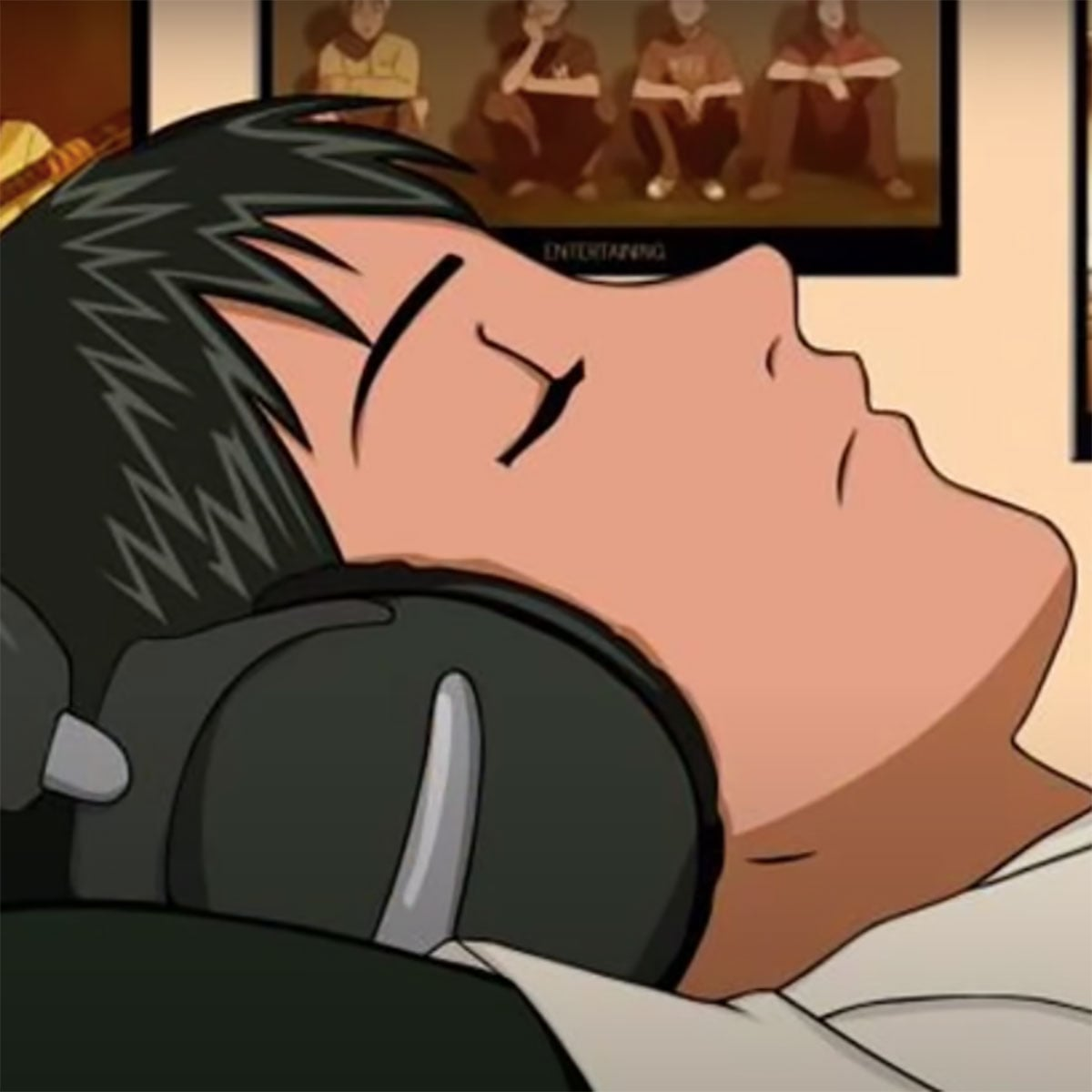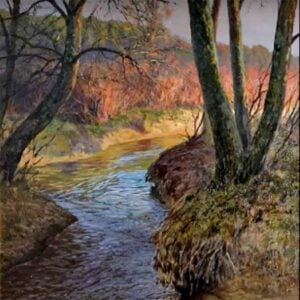2 December 2020
7
01:43
-5
• ##### TEMPO
190
0
(0)

fh h| [wtsf0]| [wt0]| [wt0]fh
[utple] k [utpk] jj[utp] h [utpf] g
[ywrj0] hh[ywr]|
[ywrfa]| [ywr] hh[tqihe] g [tihe] j
[urfW0] d [urfW] g [utpge] ff[utp]|
[utspofe]| [utpo]|
[wutp]| [utp]|
[yqe9]| [yqed] f
[tqige] f [tied] s
[wtg0]|f[wt0] d [wts0]| [wt0]|
[wtg0]| [wta0]a|
[sg8] [wtg0] g [wth0] [g8] [wtf0] ds[wt0]
[ga7] [wtg0] g [wth0] [g7] [wtf0] ds[wt0]
[g^P] [wtg0] g [wth0] [g^] [wtf0] ds[wt0]
[qj] [tie]| [tie]
[qp] [tie] i [tie]u[j9]
[yqje] j [yqje]
9 [yqhe] g [th8]
w [rf7]| [utpd]
e [utp] d [utpf]
[tqige] f [tied] s
[tie] a [tife] d
[ws]tyo|
[wa]ryo|aa|
[sg8] g [wtg0] h [wtg0] f [wtd0]s|
[ga7] g [wtg0] h [wtg0] f [wtd0]s|
[g^P] g [wtg0] h [wtg0] f [wtd0]s|
[qj] [tie]| [tie]
[qp] [tie] i [tie]u
[j9] [yqje] j [yqje]
9 [yqhe] g [th8]
w [rf7]| [utpd]
e [utp] d [utpf]
[tqige] f [tied] s
[tie] a [tife] d [ywrod]|
ds[tqigW]|
fd[ywrof]|
ds[tqigW] [tqiW] fh h| [wtb0]|
[wvt0]| [xwtf0] h
[utple] k [utpk] jj
[utp] h [utpf] g
[ywrj0] hh[ywrc] z
[yxwr] c [ywr] v
[tqihe] g [tihe] j
[urfW0] d [urfW] g
[utpge] ff[utpg]c|
[utpoe]| [utpoh]v|
[wutp]| [utpj]b|
[yqe9]| [yqed] f
[tqige] f [tied] s
[ywra] [ywr] fh h| [wtsf0]| [wt0]| [wt0]fh
[utple] k [utpk] jj[utp] h [utpf] g
[ywrj0] hh[ywr]|
[ywrfa]| [ywr] hh[tqihe] g [tihe] j
[urfW0] d [urfW] g [utpge] ff[utp]|
[utspofe]| [utpo]|
[wutp]| [utp]|
[yqe9]| [yqed] f
[tqige] f [tied] s

Rate This Music Sheet:

Average rating 0 / 5. Vote count: 0

No votes so far! Be the first to rate this music sheet.

Thank you for rating this song!

If you have any specific feedback about how to improve this music sheet, please submit this in the box below.

Follow Me (Beck) is a song by Beat Crusaders. Use your computer keyboard to play Follow Me (Beck) music sheet on Virtual Piano. This is an Intermediate song and requires a lot of practice to play well. The recommended time to play this music sheet is 01:43, as verified by Virtual Piano legend, Arda. The song Follow Me (Beck) is classified in the genres: Manga, Songs From TV, Japan on Virtual Piano. You can also find other similar songs using Anime.

## Related

•8 w y w u||| 8 w i w u||| 8 w y w u||| 8 w i w u||| [f8] w y w u| g| [d9] w y w t| s| [s4] 8 q e t| d| [f8] w t u o||| [fe] u p s| h| [qj] t i p| g| [f8] w t u| g| [wd] y o a||| [qj] t i p k| j [h0] r u o f| df[d9] e y i s| h| [f8] w t l k h f h [qj] t i p k| j [h0] r u o zx| x[ql] t [ki] p j| k l [zw] y o a x||| [f8] w y w u| g| [d9]| y w t| s| [s4]| q e t| d| f w t u o||| [fe] u p s| h| [qj] t i p| g| [f8] w t u| g| [wd] y o a f| d [s8] w t u o||| 8 w t u o| u| 8
Level: 4
Length: 01:46
Easy

#### Yasuharu Takanashi

•[tYW] OoO s Y O [wYE] POP S Y P s Ww[sWHD] t ( [sWD] [SP] EW[hE] T ( [hSPE] [OHD] tE[tD] Y W [tPD] [qOD] Hh[iH] g s D [SP] EW[HE] T w [SOE] [oS(] JH[YJ] h S [oSJ] [sO] WwWD[tg]h[qH]g[dW]g[P9] wqwd[ED]g[h(]D[ws]D[O8]P[qo][P(][qO]s[dW]D[g9]d[qP]d[o^]O[i(][O9][o(]P[ws]d[D8]s[O(]s[i%]D[qd][D(][qO]D[dW]D[i9]D[qd]D[o^]D[wd][qD][wP]D[dE]D[o(]D[wd]D[O8]D[WS][wD][sW]D[tP]D[qO]D[oW]D[i9]D[qo]D[^O]d[D9]g[o(]P[O8]s[P5]D[d4]s[P5]O[P%]s[yo^]ioi YY (9[oPD(] w ^ [oP(] [iO] q([qd] W ^ [qidO] [woYPD] YyY [o(]q[wE]q[wY](E [i^]Yi [^O]8[E9]8[i9]^[o(] [[email protected]]OP [TD]t[oE]t[WP]E[wY]W[qo][wi][o(]q[P]([Y8][o^]8[t%] YTY [OG]g[tD]g[YS]D[sW]S[tP][sE][tO]P[YI]O[iW]I[tY]i[qT] SsSW[gE]t[TP]E[wS]E[o(] sPsw[WD]E[tO]W[sq]W[i]([P8][O(][P]q[wS]W[oE]w[P(]w[Y8][^O][o][O8]([sq]w[iW]q[O]q[^T]W[wP][WO][P]W[wS]W[o^]W[wP]W[Y8]W[ws][WP][s(]W[wD]W[O8]W[ws]W[i]W[SQ][sW][qS]W[g(]W[P]W[S8]W[o^][P8]([Y]q[o(][O8](q(o[qO][P][so(]PsP[s]PsP( [[email protected]] [O%] DS[WD] s o P [iO]POq([qD] W I[qi]Y[^T] Ss[WS] P i O [oY]Oo([S(] w 8i[Y(]T[t%][sW][wP][sW][qO][sW][o(][sW][i][sW][Y8][sW][^T][SE][sW][SE][wP][SE][qO][SE][o(][SE][i][SE][Y8] [tP]OPOoO [sq]w[iW]w[WO]q[SE] [^O]oOoio [P(]q[wY]q[wo]([sW] [iTO*] [YOE(] * [o(] @ [tYO%]

Level: 6
Length: 01:02
Intermediate

#### Johann Sebastian Bach

•s f s f s f s f
[s8] f [wts] f [s6] f [se0] f
[a30] f [raW] f a [sq4] s a
[tse] f [s8] f [wts] f [s6] f
[se0] f [a30] f [raW] f a [sq4]
s a [tse] s [f1] f s [w0]
s [a6] a u 0 3 [W0] 4
[qpe] a s d [f1] f s [w0]
s [a6] a p [e0] a 3 [W0]
[s4] [qe] a [s1] s a [w0] a
[p6] p a [e0] p [f3] d s
[W0] p [f4] d [sqe] 8 f [wt]
f [p6] [e0]  f [rW] f [qp4]
[tge] 8 f [wt] f [p6] [e0] 
[rW] [q4] [te] s [f1] f s [w0]
s [a6] a u 0 3 [W0] 4
[qpe] a s d [f1] f s [w0]
s [a6] a p [e0] a 3 [W0]
[s4] [qe] a [s1] s a [w0] a
[p6] p a [e0] p [f3] d s
[W0] p [f4] d [sqe] 8 f [wt]
f [p6] [e0]  f [rW] f [qp4]
[tge] 8 f [wt] f [p6] [e0] 
[rW] [q4] [te] [h8] [wt] h g f
d [f6] [e0] f [d30] [rW] d [sq4]
a p a [tse] d f g [h8]
[wt] h g f d [f6] [e0] f
[d30] [rW] d [sq4] a p a [tse]
d f g [h8] [wtsl] [ka6] [e0] 
[rW] [sql4] [te] 8 [wtsl] [ka6] [e0] 
[rW] [q4] [tW] [s8] f [wts] f [s6]
f [se0] f [a30] f [raW] f a
[sq4] s a [tse] f [s8] f [wts]
f [s6] f [se0] f [a30] f [raW]
f a [sq4] s a [tse]

Level: 5
Length: 02:18
Intermediate